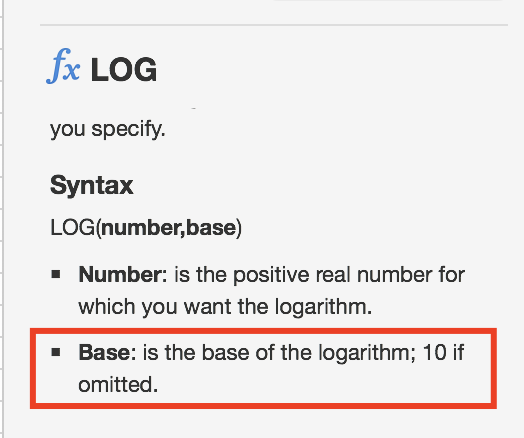# Cross-Entropy: using natural log vs log10 and sum vs mean

In the Machine Learning course, lesson 8, around the 1 hour mark, Jeremy shows us how to calculate binary cross-entropy in Python. The code used in provided below, which just takes the mean of the logged predictions.

``````np.mean(-(y * np.log(p) + (1-y) * np.log(1-p)))
``````

Based on my understanding, np.log( ) is taking the natural log and as the code then shows, we take the mean of those logs (np.mean). So far so good.

But, when Jeremy switches to Excel, we appear to be using log10 (see Excel help docs screenshot below) and we are summing the results vs taking the mean.This results in two different loss scores for the same dummy data:

0.16425 —> Python code using natural log and mean
0.28533 —> Excel code using log10 and sum

Doing some reading online, and based on my very limited understanding, it appears we should:

1. Use the natural log; and
2. Take the sum.

Based on everything I have read, the natural log is the way to go, but still a little confused as to taking the average or the sum.

Is anyone able to confirm which of the following methods of calculating cross-entropy is correct:

1. Calculate using the mean of the natural logged predictions
2. Calculate using the sum of the natural logged predictions
3. Both are valid methods

Thanks in advance for anyone who can assist.

Todd